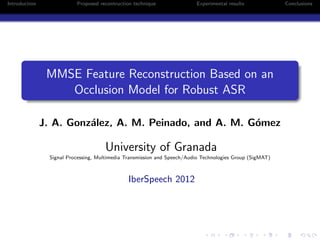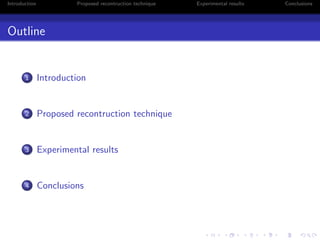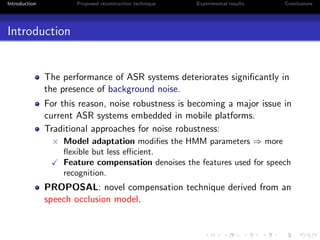Successfully reported this slideshow.

# Iberspeech2012

1

Share×
1 of 15
1 of 15

# Iberspeech2012

1

Share

## More Related Content

### Related Books

Free with a 14 day trial from Scribd

See all

### Related Audiobooks

Free with a 14 day trial from Scribd

See all

### Iberspeech2012

1. 1. Introduction Proposed recontruction technique Experimental results Conclusions MMSE Feature Reconstruction Based on an Occlusion Model for Robust ASR J. A. Gonz´alez, A. M. Peinado, and A. M. G´omez University of Granada Signal Processing, Multimedia Transmission and Speech/Audio Technologies Group (SigMAT) IberSpeech 2012
2. 2. Introduction Proposed recontruction technique Experimental results Conclusions Outline 1 Introduction 2 Proposed recontruction technique 3 Experimental results 4 Conclusions
3. 3. Introduction Proposed recontruction technique Experimental results Conclusions Introduction The performance of ASR systems deteriorates signiﬁcantly in the presence of background noise. For this reason, noise robustness is becoming a major issue in current ASR systems embedded in mobile platforms. Traditional approaches for noise robustness: × Model adaptation modiﬁes the HMM parameters ⇒ more ﬂexible but less eﬃcient. Feature compensation denoises the features used for speech recognition. PROPOSAL: novel compensation technique derived from an speech occlusion model.
4. 4. Introduction Proposed recontruction technique Experimental results Conclusions Occlusion Model (I) The mismatch function for the log-Mel domain can be approximated by: y ≈ log(ex + en ) This model can be rewritten as, y ≈ max(x, n) + ε(x, n) where ε(x, n) = log 1 + emin(x,n)−max(x,n) " (x ; n )" (x ; n ) 23 15 7 0.6 0 0.5 1.0 1.5 2.0 2.5 3.0 Time (s) 0.4 0.2 12 0 Clean 23 15 7 Noisy (0 dB) 23 15 7 12 5 Log-Max 23 15 7 12 5 0 0.01 0.02 0.03 0.04 0.05 0.06 0.07 0.08 -0.4 -0.2 0 0.2 0.4 0.6 Probability ε(x, n) Distribution of ε(x, n) in Aurora2
5. 5. Introduction Proposed recontruction technique Experimental results Conclusions Occlusion Model (II) Hence, ε(x, n) can be safely ignored from the above model: y ≈ max(x, n) (1) This equation will be referred to as the speech occlusion model (a.k.a. Log-Max approximation). According to (1), the noisy feature vector can be rearranged into y = (yr , yu): Reliable features (xr ≈ yr ), i.e. speech is unaﬀected. Unreliable features (−∞ ≤ xu ≤ yu): speech is masked by noise. PROBLEM: How do we estimate the unreliable/reliable features? Missing data techniques (MDT) use missing-data masks. Our proposal uses noises estimates to this end.
6. 6. Introduction Proposed recontruction technique Experimental results Conclusions Proposed Technique: Visual Analogy MMSE estimation ASR Noisy input Noise estimate Source model Enhanced input
7. 7. Introduction Proposed recontruction technique Experimental results Conclusions MMSE Estimation of Occluded Features (I) The MMSE estimate of the clean feature vector is given by: ˆx = E[x|y] = x xp(x|y)dx Source model: clean speech GMM λX with M gaussians. Noise estimates: p(nt|λN,t) = NN(nt; µN,t, ΣN,t). Therefore, the MMSE estimate can be ﬁnally expressed as (time dependency is omitted), ˆx = M k=1 P(k|y, λX , λN) x xp(x|y, k, λX , λN)dx E[x|y,k,λX ,λN ]
8. 8. Introduction Proposed recontruction technique Experimental results Conclusions MMSE Estimation of Occluded Features (II) Posterior computation: the corrupted speech likelihood can be computed as the product of the following probabilities: p(yi |k) = pX (yi |k)CN(yi ) + pN(yi )CX (yi |k) Similarly, the partial estimates are given by, E [xi |yi , k] = wk i yi + (1 − wk i )˜µk X,i wk i : speech presence probability, i.e. wk i = P(xi > ni |k). yi : clean speech estimate for high SNRs. ˜µk X,i : estimate for the case of total occlusion ⇒ xi ∈ (−∞, yi ].
9. 9. Introduction Proposed recontruction technique Experimental results Conclusions Comparison with related techniques Missing-data techniques (MDT) are also based on the speech occlusion model. MDT assume that a priori knowledge about the feature reliability is provided by a missing-data mask m. m: either binary (mi = 1 ⇔ yi reliable, mi = 0 ⇔ yi unreliable) or soft (mi ∈ [0, 1]). Then, the terms required by the MMSE estimator are: p(yi |k) = mi pX (yi |k)C(yi ) + (1 − mi )pN(yi )CX (yi |k) E[xi |yi , k] = mi yi + (1 − mi )˜µk X,i
10. 10. Introduction Proposed recontruction technique Experimental results Conclusions Experimental setup The proposed technique is evaluated on the Aurora2 and Aurora4 databases. Speech features: 13 MFCCs + ∆ + ∆2 + CMN. Clean speech GMM with 256 components and diagonal covariances. Noise estimation: linear interpolation of the means for the N ﬁrst and last frames (NAurora2 = 20, NAurora4 = 40). ΣN ﬁxed for the whole utterance. Mask computation: Binary masks: SNR estimates are thresholded (0 dB). Soft masks: see equation in the next slide.
11. 11. Introduction Proposed recontruction technique Experimental results Conclusions Illustrative example Clean Noisy (0 dB) Noise Enhanced speech Estimated mask 23 15 7 12 0 23 15 7 12 5 23 15 7 12 5 23 15 7 11 3 23 15 7 1 0 0.5 1.0 1.5 2.0 2.5 3.0 Time (s) Utterance (eight six zero one one six two) corrupted by subway noise at 0 dB. Feature reliability mask is computed as follows: mi = M k=1 P (k|y, λX , λN) wk i (2)
12. 12. Introduction Proposed recontruction technique Experimental results Conclusions Aurora2 results 10 20 30 40 50 60 70 80 90 100 -5 0 5 10 15 20 Clean WAcc(%) SNR (dB) Baseline Oracle BMD SMD SRO Average results for Sets A, B, and C. Oracle: MDT with perfect knowledge of the feature reliability. MDT: BMD (binary masks) and SMD (soft masks). SRO (proposed technique) outperforms BMD and SMD.
13. 13. Introduction Proposed recontruction technique Experimental results Conclusions Aurora4 results T-01 T-02 T-03 T-04 T-05 T-06 T-07 Avg. Baseline 87.69 75.30 53.24 53.15 46.80 56.36 45.38 59.70 Oracle 87.69 86.74 84.46 84.44 83.19 85.90 82.38 84.97 BMD 86.96 80.78 58.47 52.74 59.63 56.14 61.42 65.16 SMD 87.52 83.65 66.62 63.78 63.48 69.19 65.31 71.36 SRO 87.54 83.28 69.23 64.49 64.88 70.63 66.93 72.43 Oracle is the recognition upper bound that can be achieved. BMD vs. SMD: soft masks are better. SRO vs. SMD: quite similar to each other, but SRO is better.
14. 14. Introduction Proposed recontruction technique Experimental results Conclusions Conclusions We have proposed a novel technique for log-Mel feature reconstruction. The technique resembles previous work on missing-data reconstruction. Contrary to MDT, our proposal requires no missing-data mask, but noise estimates. The proposed technique can be alternatively considered as a mask estimation technique. The experimental results have shown the superiority of our proposal over MDT.
15. 15. Introduction Proposed recontruction technique Experimental results Conclusions Thank you!